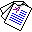# On Abductive Equivalence

## Katsumi Inoue and Chiaki Sakama

Lorenzo Magnani (ed.), Model-Based Reasoning in Science and Engineering. Cognitive Science, Epistemology, Logic, pages 333-352, College Publications, London, 2006.

## Abstract

We consider the problem of identifying equivalence of two knowledge bases which are capable of abductive reasoning. Here, a knowledge base is written in either first-order logic or nonmonotonic logic programming. In this work, we will give two definitions of abductive equivalence. The first one, explainable equivalence, requires that two abductive programs have the same explainability for any observation. Another one, explanatory equivalence, guarantees that any observation has exactly the same explanations in each abductive framework. Explanatory equivalence is a stronger notion than explainable equivalence, and in fact, the former implies the latter. In first-order abduction, explainable equivalence can be verified by the notion of extensional equivalence in default theories. In nonmonotonic logic programs, explanatory equivalence can be checked by means of the notion of relative strong equivalence. We also discuss how the two notions of abductive equivalence can be applied to extended abduction, where abducibles can not only be added to a program but also be removed from the program to explain an observation.Full Paper (PDF 140K)